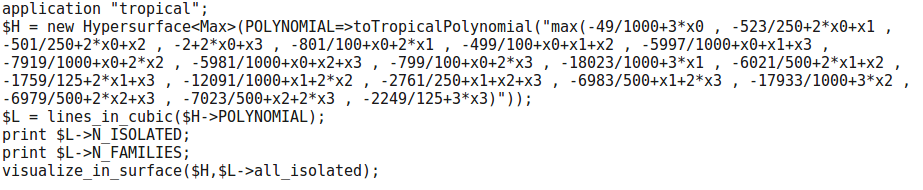# Sampling from a $$p$$-adic manifold

Yassine El Maazouz and Enis Kaya: Sampling from $$p$$-adic manifold

Abstract: We give a method for sampling points from an algebraic manifold (affine or projective) over a local field with a prescribed probability distribution. In the spirit of the previous work by Breiding and Marigliano on real algebraic manifolds, our method is based on slicing the given variety with random linear spaces of complementary dimension. We also provide an implementation of our sampling method and discuss a few applications. For example, we sample from algebraic $$p$$-adic matrix groups and modular curves.This repository includes an implementation of the sampling method in the special case where we sample from $$\mathcal{O}$$-valued points of the manifold $$X$$.

Project page created: 15/06/2022.

Project contributors: Yassine El Maazouz and Enis Kaya.